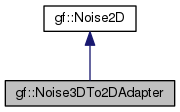Gamedev Framework (gf)  0.10.0 A C++14 framework for 2D games

An adapter that make a 2D noise from a 3D noise. More...

#include <gf/Noises.h>[legend]

## Public Member Functions

Noise3DTo2DAdapter (Noise3D &noise, Vector3d normal=Vector3d(0.0, 0.0, 1.0), Vector3d point=Vector3d(0.0, 0.0, 0.0))
Constructor. More...

virtual double getValue (double x, double y) override
Take a 2D noise value. More...Public Member Functions inherited from gf::Noise2D
virtual ~Noise2D ()
Virtual destructor. More...

double operator() (double x, double y)
Take a 2D noise value. More...

## Detailed Description

An adapter that make a 2D noise from a 3D noise.

The 3D point is taken on a plane defined by a normal and a point. The 3D point has the same $$x$$ and $$y$$ coordinates as the 2D point, and the plane is used to determine the $$z$$ coordinate.

By default, the $$z = 0$$ plane is used.

## Constructor & Destructor Documentation

 gf::Noise3DTo2DAdapter::Noise3DTo2DAdapter ( Noise3D & noise, Vector3d normal = Vector3d(0.0, 0.0, 1.0), Vector3d point = Vector3d(0.0, 0.0, 0.0) )

Constructor.

Parameters
 noise The original 3D noise normal The normal of the 3D plane point The point of the 3D plane

## ◆ getValue()

 virtual double gf::Noise3DTo2DAdapter::getValue ( double x, double y )
overridevirtual

Take a 2D noise value.

Parameters
 x The x coordinate of the noise value y The y coordinate of the noise value
Returns
The noise value

Implements gf::Noise2D.